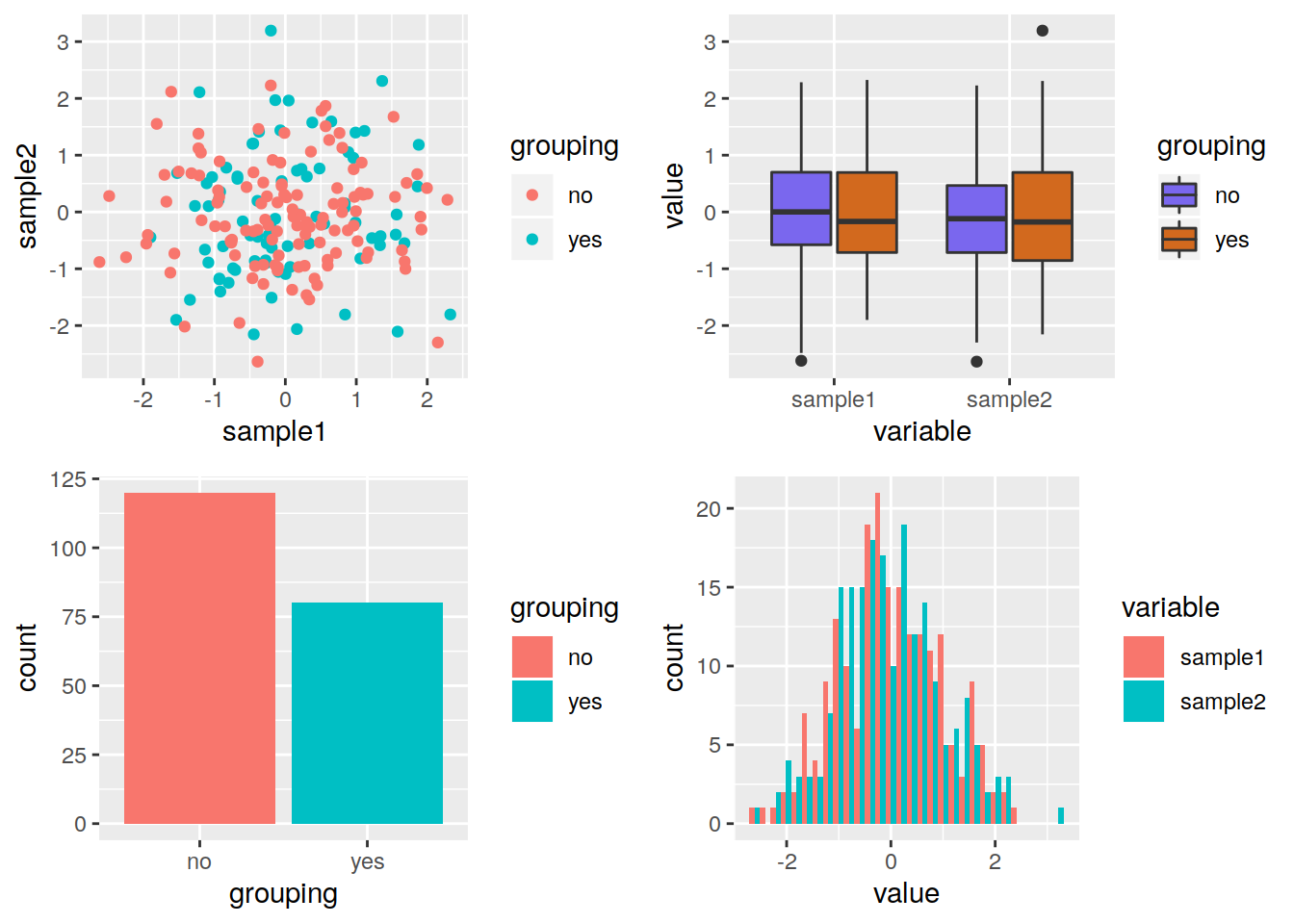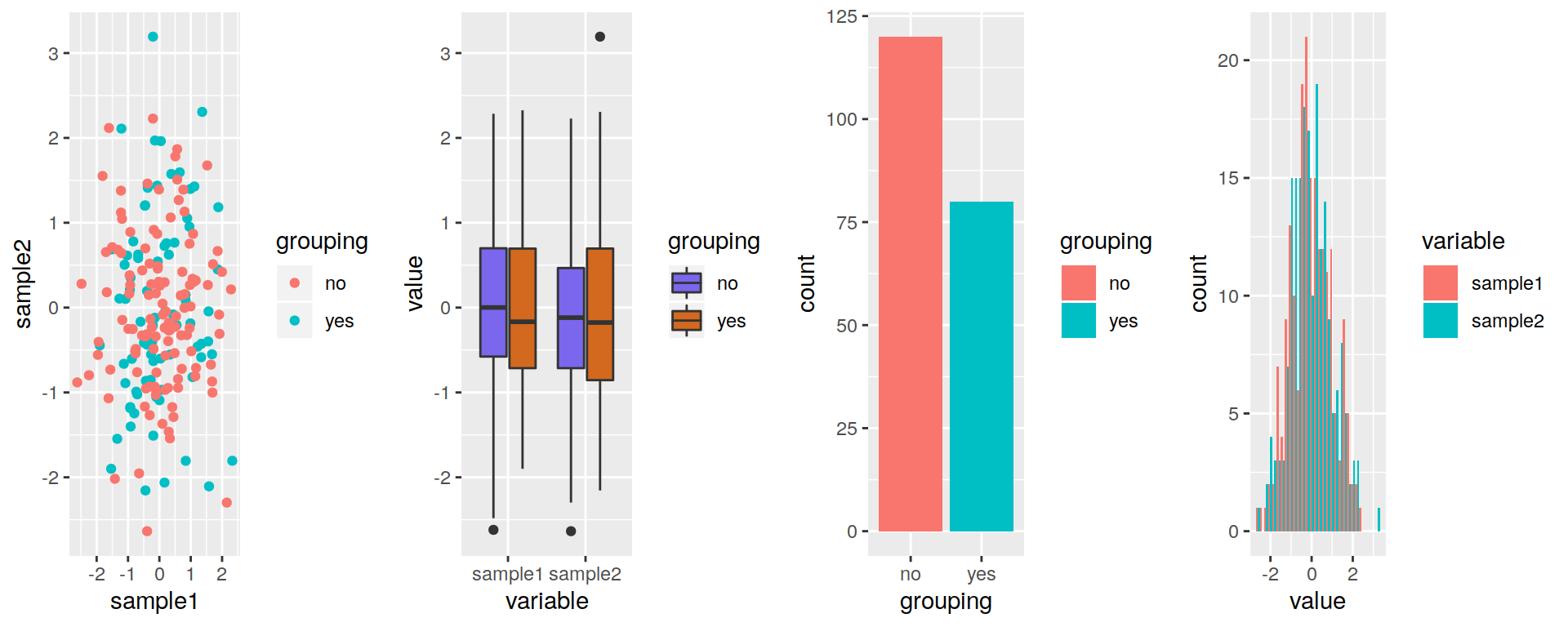## 19.8 Saving plots in files

• The same as for regular plots applies:
``````png("myggplot.png")
p
dev.off()``````
• You can also use the ggplot2 ggsave function:
``````# By default, save the last plot that was produced
ggsave(filename="lastplot.png")

# You can pick which plot you want to save:
ggsave(filename="myplot.png", plot=p)

# Many different formats are available:
# "eps", "ps", "tex", "pdf", "jpeg", "tiff", "png", "bmp", "svg", "wmf"
ggsave(filename="myplot.ps", plot=p, device="ps")

# Change the height and width (and their unit):
ggsave(filename="myplot.pdf",
width = 20,
height = 20,
units = "cm")``````
• You can also organize several plots on one page
• One way is to use the gridExtra package:
• ncol, nrow: arrange plots in such number of columns and rows
``install.packages("gridExtra")``
``````# load package
library(gridExtra)
# 2 rows and 2 columns
grid.arrange(pscat, pbox_fill, pbar, phist, nrow=2, ncol=2)````````````# 1 row and 4 columns
grid.arrange(pscat, pbox_fill, pbar, phist, nrow=1, ncol=4)``````Combine ggsave and grid.arrange:

``````myplots <- grid.arrange(pscat, pbox_fill, pbar, phist, nrow=1, ncol=4)

ggsave(filename="mygridarrange.png", plot=myplots))``````